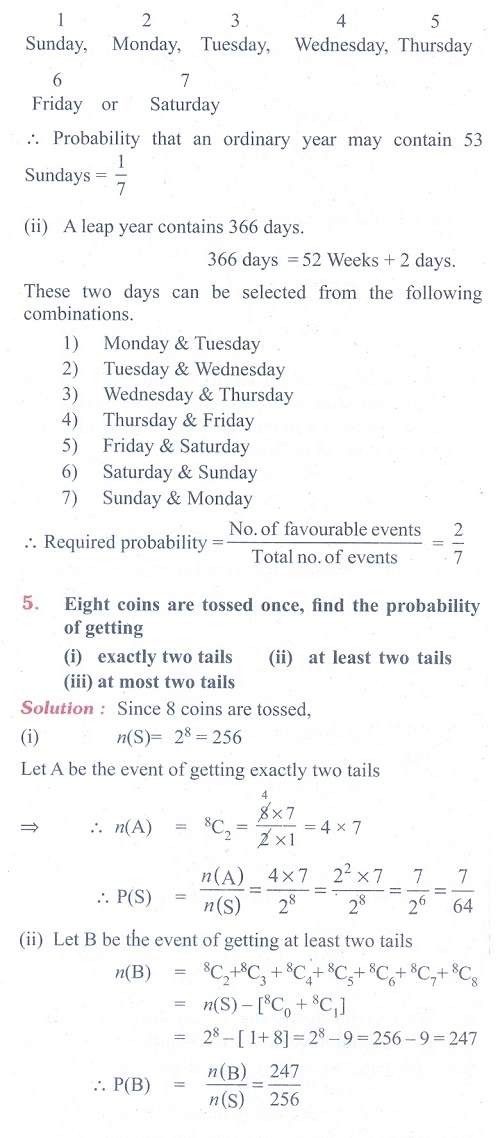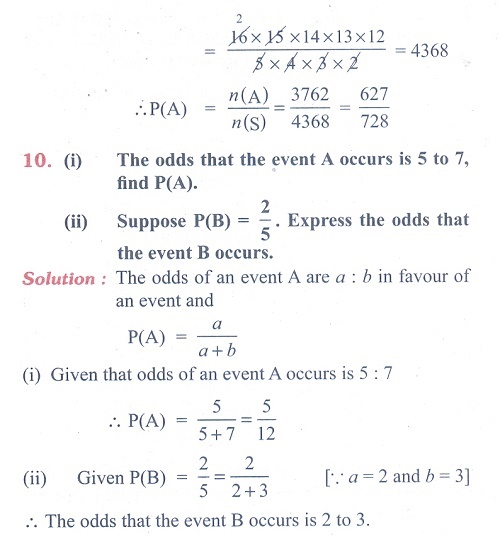Home | | Maths 11th std | Exercise 12.1: Odds - Probability Theory

# Exercise 12.1: Odds - Probability Theory

Maths Book back answers and solution for Exercise questions - Mathematics : Introduction to Probability Theory

### EXERCISE 12.1

(1) An experiment has the four possible mutually exclusive and exhaustive outcomes A, B, C, and D. Check whether the following assignments of probability are permissible.

(i) P(A) = 0.15, P(B) = 0.30, P(C) = 0.43, P(D)= 0.12

(ii) P(A) = 0.22, P(B) = 0.38, P(C) = 0. 16, P (D) = 0.34

(iii) P(A) = 2/5 , P(B) = 3/5 , P(C) = ŌłÆ 1/5 , P(D) = 1/5

(2) If two coins are tossed simultaneously, then find the probability of getting

(i) one head and one tail (ii) at most two tails

(3) Five mangoes and 4 apples are in a box. If two fruits are chosen at random, find the probability that (i) one is a mango and the other is an apple (ii) both are of the same variety.

(4) What is the chance that (i) non-leap year (ii) leap year should have fifty three Sundays?

(5) Eight coins are tossed once, find the probability of getting

(i) exactly two tails (ii) at least two tails (iii) at most two tails

(6) An integer is chosen at random from the first 100 positive integers. What is the probability that the integer chosen is a prime or multiple of 8?

(7) A bag contains 7 red and 4 black balls, 3 balls are drawn at random. Find the probability that (i) all are red (ii) one red and 2 black.

(8) A single card is drawn from a pack of 52 cards. What is the probability that

(i) the card is an ace or a king

(ii) the card will be 6 or smaller

(iii) the card is either a queen or 9?

(9) A cricket club has 16 members, of whom only 5 can bowl. What is the probability that in a

team  of 11 members at least 3 bowlers are selected?

(10) (i) The odds that the event A occurs is 5 to 7, find P(A).

(ii) Suppose P(B) = 2/5 . Express the odds that the event B occurs.Tags : Problem Questions with Answer, Solution | Mathematics , 11th Mathematics : UNIT 12 : Introduction to Probability Theory
Study Material, Lecturing Notes, Assignment, Reference, Wiki description explanation, brief detail
11th Mathematics : UNIT 12 : Introduction to Probability Theory : Exercise 12.1: Odds - Probability Theory | Problem Questions with Answer, Solution | Mathematics